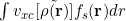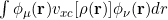# psi4.core.VBase and nblocks() issue

Hello,
I am playing around with the psi4.core.Vbase class. My aim is to evaluate such integral, namely the projection of the exchange-correlation potential on an arbitrary set of functions. The result should be a column vector (of length s)
The integral itself does not pose any particular challenges since is quite similar to the integralas shown in the “/Tutorials/04_Density_Functional_Theory/4b_LDA_kernel.ipynb” notebook

Nevertheless, I am facing some problems when I try to get some info about the functional and the n. of blocks
The folling simple python script ends in a segfault. [In the Psi4NumPy notebook, the potential is retrieved from wfn (i.e wfn.V_potential()), but I would prefer to avoid a complete scf run on top of that]

``````import numpy as np
import psi4

from pkg_resources import parse_version
mol = psi4.geometry("""
H 0.0 0.0 -0.3677
H 0.0 0.0  0.3677
symmetry c1
""")
#O
#H 1 1.1
#H 1 1.1 2 104
basisname = 'cc-pvdz'
psi4.set_options({'basis':        basisname,
'scf_type':         'direct',
'puream':              'False',
'e_convergence':    1e-8,
'd_convergence':    1e-8})

# build basisset
test_basis = psi4.core.BasisSet.build(mol,'ORBITAL',basisname,puream=-1)

nbf = test_basis.nbf()

D = np.random.rand(nbf,nbf)

D = 0.5*(D + D.T)

restricted = True
if parse_version(psi4.__version__) >= parse_version('1.3a1'):
build_superfunctional = psi4.driver.dft.build_superfunctional
else:
build_superfunctional = psi4.driver.dft_funcs.build_superfunctional
sup = build_superfunctional('BLYP', restricted)  # the superfunctional
sup.set_deriv(2)
sup.allocate()
vname = "RV"
if not restricted:
vname = "UV"
potential=psi4.core.VBase.build(test_basis,sup,vname)
# intialize() missing
potential.initialize()
#get some info
point_func = potential.properties()